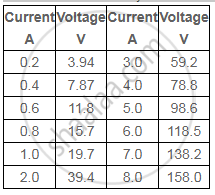Share

# What Conclusion Can You Draw from the Following Observations on a Resistor Made of Alloy Manganin? - CBSE (Science) Class 12 - Physics

ConceptResistivity of Various Materials

#### Question

What conclusion can you draw from the following observations on a resistor made of alloy manganin?#### Solution

It can be inferred from the given table that the ratio of voltage with current is a constant, which is equal to 19.7. Hence, manganin is an ohmic conductor i.e., the alloy obeys Ohm’s law. According to Ohm’s law, the ratio of voltage with current is the resistance of the conductor. Hence, the resistance of manganin is 19.7 Ω.

Is there an error in this question or solution?

#### APPEARS IN

NCERT Solution for Physics Textbook for Class 12 (2018 to Current)
Chapter 3: Current Electricity
Q: 17 | Page no. 129

#### Video TutorialsVIEW ALL 

Solution What Conclusion Can You Draw from the Following Observations on a Resistor Made of Alloy Manganin? Concept: Resistivity of Various Materials.
S